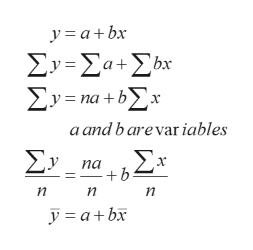# Use the general equation for the least square regression line to show that this line always passes through the point (x,y) * bars above the x and y.That is, set x=x(with a bar above the x) and show that the line predicts that y=y (with a bar above the y).

Question
39 views

Use the general equation for the least square regression line to show that this line always passes through the point (x,y) * bars above the x and y.That is, set x=x(with a bar above the x) and show that the line predicts that y=y (with a bar above the y).

check_circle

Step 1

The linear regression equation always passes through (x, y)

y =a + bx

Let there be two variables: x & y.

y – Regression or dependent variable or explained variable
x – Independent variable or predictor or explanatory variable

Step 2

On minimizing the least squares equation, here is what we ...help_outlineImage Transcriptionclosey= a+bx ΣΥ-Σα+Συ ΣΥ-m+bΣ: a and b arevar iables ΣΥ па +b η n y =a+bx fullscreen

### Want to see the full answer?

See Solution

#### Want to see this answer and more?

Solutions are written by subject experts who are available 24/7. Questions are typically answered within 1 hour.*

See Solution
*Response times may vary by subject and question.
Tagged in

### Statistics